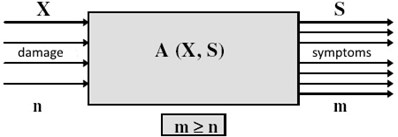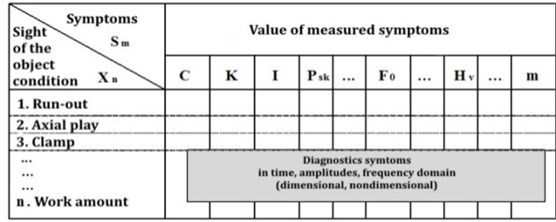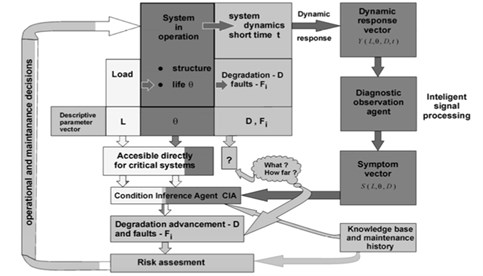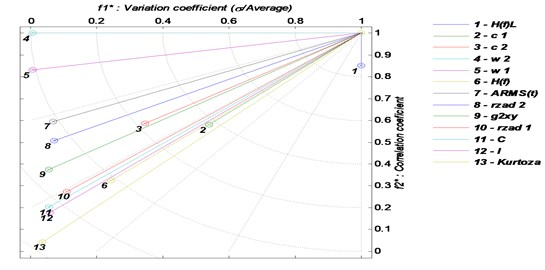Published: 09 October 2015

# Vibration assessment of the consumption of a technical object

Bogdan Żółtowski1
Marcin Łukasiewicz2
1, 2UTP University of Science and Technology, Bydgoszcz, Poland
Corresponding Author:
Marcin Łukasiewicz
Views 10

#### Abstract

Machines in operation are subject to degradation controlled by diagnostic methods. The vibrodiagnostics is one of the machine condition description methods – understood as the organized set of methods and means to the technical state estimation (their causes, evolution and consequence) of technical systems, with utilization of vibration processes or the noise signal. Obtaining information on the condition and its processing for the purposes of legitimate exploitation decisions is elaborated on in many aspects, considered as new herein. This involves information selection, dedicated diagnostic systems, the system of agreeing on decisions, and cause-effect modelling. Selected aspects of this subject are discussed also in this publication.

## 1. Introduction

Every technical device in the given moment is in certain definite state. The most general technical state of an object, machine or vehicle can be described as a set of parameter values that define the given object in a given moment of time t. The time sequences of these states could be considered as the time of the device existence. It inevitably lead the destructive influences of external extorting and internal factors to the machine condition change. The use of technical diagnostics methods makes possible to qualification of current technical state of studied object, machine and vehicle possible [1-6].

The present development of automation and computer science in range of technical equipment and software creates new possibilities of realization of diagnosing systems and monitoring technical condition of more folded mechanical constructions. These new possibilities are connected with the new constructions of intelligent sensors, module software and the modules of transport and data exchange [7-12]. Modern machines features are described by their: functionality, safety, reliability, readiness, mobility and exploitation susceptibility. The main aim of applying the machines diagnostics is the qualification of object condition based on the generated diagnostics signals (symptoms) diagnostic and comparing them with nominal values.

## 2. The basis of machine vibrodiagnostics

The vibrodiagnostics is one of the machine condition description methods - understood as the organized set of methods and means to the technical state estimation (its causes, evolution and consequence) of technical systems, with utilization of vibration processes or the noise signal [13-16].

The vibroacoustics – is the discipline of knowledge focusing on all vibration processes, acoustic and pulsating processes setting in nature, technique and machines. The vibroacoustics processes are not only harmful but they are also parasitic processes. If applied suitably, they can be a good carrier of the energy which can be used to the realization of varied technological processes. The main tasks of vibroacoustics:

• the identification of vibroacoustics energy sources,

• the study of the propagation ways of the vibroacoustics energy in real constructions and environment,

• the study of the vibroacoustics energy control methods (emission, propagation) in machines and environment,

• utilization of the vibroacoustics signals for the aims of technical diagnostics,

• vibroacoustics synthesis of machines and objects, led for the obtainment of the optimum vibroacoustics activity. The active uses of vibroacoustics [13-16].

Onto investigations of vibroacoustics process it use energy methods, discrete methods, and in the peculiarity the finite element method, the modal analysis methods and the analysis method of the acoustic power flow [17-19]. In the diagnostic use, vibroacoustics signal independently from mold in which was introduced to the diagnostic system, it could be processed to the sets of parameters whose values are the basis of the diagnostic objects conditions sights description. The choice of these parameters fundamentally influence on the efficiency of diagnosis process that often is deficient of circumstances for this choice optimization. The general essence of the vibroacoustics diagnostics bases on the search of relationships among the condition of machine ${X}_{n}$, and generated vibroacoustics signals ${S}_{m}$, with the omission of different external influences, as it introduces on Fig. 1 [13-16].

Fig. 1The observation model of machine state X basis on symptoms SBasis so formulated observation model we could create the matrix of observation in which possible damages ($n$) were taken down from one side represented by technical state sights that imitating evolving of damage, and from second side we receive the aggregate of symptoms ($m$), characteristic for the condition of development damage in the moment of the symptoms measurement. The observation matrix of measured symptoms was introduced on Fig. 2.

Fig. 2The observation matrix of measured symptomsBasis on the observation matrix we can affirm, that many symptoms can inform about one failure and solution of the diagnostic problem requires the fulfilments of the condition: $m\ge n$. Applied operator A, binding the sight of the object state $X$ and his symptoms $S$ after identification, lets on the basis of measured symptoms infer about the object state $X$.

## 3. Information system of identification tests

The assessment of the technical condition of machinery with the use of physical processes generated by it requires obtaining relevant information on the condition and associating functional parameters of the evaluated object properly, including a set of measurements and an evaluation of the initial processes. The development of virtual techniques gives rise to numerous new solutions for the modelling, simulating, collecting, and processing of diagnostic information. Some of them were briefly presented signal processing, statistical optimization of results, and diagnostic deduction in making exploitation decisions. The previous is possible due to the algorithm named Condition Inference Agent – CIA which was incorporated in the design of the SPD (Fig. 3). This algorithm was designed in European diagnosis institutes for the technical critical systems of operation, in order to increase the maintenance indicators of reliability and availability. To develop this diagnosis tool, there were used different methodologies that group several modern and effective methods in the diagnosis tasks, which go from the selection of measurement points, through the method of evaluation until the utilization of an optimized forecast method. The aim of this article is to shows the different methodologies previously pointed out [1, 2, 7, 10, 14, 18].

Fig. 3Flow of information and processing in a system of operation and a subsystem of condition of intelligent monitoringThe observation of progress in the wear and tear of an object is based on measuring various symptoms of the technical condition and comparing them with the allowed values established earlier – for a specific symptom and in a particular application. The process of object wear and tear is usually not one-dimensional, and the dimension of damage degree increases with the degree of machinery construction complexity. This radically improves the dimensionality of condition vectors, signal, and interference vectors. Diagnostic information available during the check-up becomes redundant, dimensionally complex, and difficult to process. This study presents the problems of information redundancy, the assessment of individual measurements of diagnostic signals, and multi-dimensional processing of diagnostic information in program research as key issues. The decision-making process consists of a series of operations, starting with obtaining information on machinery status, its gathering, and processing, until selecting and forwarding a fixed decision for implementation. In the beginning, however, three types of initial data processing need to be distinguished: data transformations, filling in the missing values, and dimensionality reduction.

Data transformations – in case of diagnostic information processing systems, most often all the features that describe analysed objects have to be numeric. In case of classification models using distance as a measurement of similarity, it is very often the case that individual features characterise a physical state on the basis of various physical quantities, having different ranges of values, and, as a result, they can have a different impact on distance. A few transformations unifying the influence of individual features to the value of distance can be applied.

Normalization – as a result of normalization, vectors with feature values from the range [0, 1] are obtained. This transformation does not take into account the value distribution of a given symptom; therefore, in the case of the appearance of several symptoms with values significantly different, most values are pressed narrow range as a result of normalization.

Standardization. The use of distribution value in single symptoms leads to a transformation known as standardization. As a result of this transformation, symptoms with an average value of $x=$ 0 are obtained, and a standard deviation $\sigma =$ 1, so that all the symptoms have an equal share with respect to the value of information.

Precision constant – takes into account the range of variation and an average value of measured parameters and provides non-dimensionality, as per the following relationship:

1
${p}_{i}=\frac{\stackrel{-}{{x}_{i}}}{{w}_{i}}.$

Symptom sensitivity ${w}_{i}$ – expressed as one number together with an average value ensures non-dimensionality and changeability range:

2
${w}_{i}=\frac{{x}_{imax}-{x}_{imin}}{\stackrel{-}{{x}_{i}}}.$

Ensuring the possibility of mutual further consideration of data obtained in measurement is an important and necessary step to make at the very beginning.

### 3.1. The engineer application “symptoms generation”

Basis on the MATLAB environment the application “Symptoms Generation” was worked out at University of Science and Technology in Bydgoszcz for vibroacoustics process analysis, serving to processing and analysis of the investigative data. The software enable processing vibroacoustics signal from amplitude of vibration in time domain to chosen estimators of vibration process as: average value, root mean square, maximal and minimal value of data set, kurtosis, skewness, standard deviation, peak factor, form factor, impulse factor, clearance factor, Rice frequency, autocorrelation, cross-correlation, covariance, coherence function, spectral density, bispectrum, bicoherence, wavelet, Wigner Ville, equivalent sound level. Basis on these estimators we could use Optimum method to optimization [9, 20].

### 3.2. Ideal point method OPTIMUM and SVD

Diagnostic signals measured in different ways reflect observation space, and in an indirect way, damage development in a machine. With the use of optimization techniques, sensitivity of measured symptoms to condition changes can be characterized based on measuring the distance from the ideal point. Distinguishing the fault is possible – according to mathematical formalism – after projecting constituent symptoms on axes $x$, $y$, $z$ respectively. The following algorithm makes statistical assessment of individually-elaborated diagnostic symptoms possible, resulting in a final ranking of their sensitivity and relevance. The following steps of this procedure include:

1. Creating an observation matrix from measured symptoms: ${s}_{1}$, ${s}_{2}$, ${s}_{3}$,…, ${s}_{m}$;

2. Statistical assessment of symptoms with the use of various criteria, i.e.:

– Symptom changeability:

3
${f}_{1}=\frac{{s}_{j}}{\stackrel{-}{y}},$

where: ${s}_{j}$ – standard deviation, $\stackrel{-}{y}$ – average value.

– Assessment of symptom sensitivity ${w}_{i}$;

– Correlation to the technical condition, ${r}_{xy}$ (determination of the correlation coefficient: symptom-condition):

4
${r}_{xy}=\frac{1}{n-1}\frac{{\sum }_{i=1}^{n}\left({x}_{i}-\stackrel{-}{x}\right)\left({y}_{i}-\stackrel{-}{y}\right)}{{\sigma }_{x}{\sigma }_{y}}.$

To make considerations and the presentation of results on the surface easier, two selected indicators of quality are sufficient.

3. Making further maximization and normalization of adopted indicators of quality signals, we obtain statistical characteristics of their sensitivity (${f}_{1}^{*},{f}_{2}^{*}$) which further allows us to determine the coordinates of an ideal point. This allows distance determination of single signal measurements from an ideal point, according to the following relation:

5
$L=\sqrt{{\left(1-{f}_{1}^{*}\right)}^{2}+{\left(1-{f}_{2}^{*}\right)}^{2}}.$

4. General sensitivity coefficients (weights) for each tested signal are determined as per the relationship:

6
${\mathrm{w}}_{j}=\frac{1}{\frac{1}{{L}_{i}}\bullet \sum _{i=1}^{n}{L}_{i}},$

where:

7
$\sum {w}_{j}=1.$

Fig. 4 shows the final result of this procedure for sample measurement data. Distance points of each measurement from an ideal point (1, 1) indicate the sensitivity of assessed signal measurements, with the points closest to (1, 1) being the best symptoms. Having highlighted statistically good symptoms, one can build cause-effect models at the stage of inferring the condition. The quality of the model depends, however, on the number of measures taken into account which indirectly, in the simplest regression models, can be evaluated with the determination of coefficient ${R}^{2}$.

Fig. 4Ideal point method outcome – OPTIMUMThe next step of identification is Singular Value Decomposition (SVD) is a numeric procedure for the multi-dimensional tracking of changes of an object’s condition. It detects developing damage and selects maximally informative condition symptoms in a given situation. The idea of SVD method was described in detail in bibliography [2, 15, 19, 21]. The possibility of rapid identification of damage while diagnosing the elements affecting the functioning of objects was the basis for the creation of “SIBI” program designed at University of Science and technology in Bydgoszcz. This program is a software implementation attempt for the following purposes: acquisition of vibration processes, their processing, testing co-dependencies of vibration processes, testing symptom sensitivity, statistical inference, and visualization of analysis results. On the market there are many CMMS systems (Computerised Maintenance Management Systems) in a wide range of prices and possibilities. An important issue for enterprise decision-makers is the choice of proper system supporting motion maintenance services .

## 4. Conclusions

This article discusses the reduction of redundancy for individual condition symptoms and for a multi-dimensional condition test. A new, simple, and effective method of sensitivity assessment for individual measurements of condition was proposed – the OPTIMUM method; moreover, the essence of the SVD method, SIBI program, and guidelines for the selection of rational commercial programs in the field of machinery exploitation were explained. Model of machine in good condition and model of the same machine after certain period of usage gives as an inference base about object waste, vibration predominant sources, that allows in the next steep the construction modernization. Selecting a method for the specificity of enterprise business depends on a number of internal or external factors. It is impossible, however, for an organization to survive without adopting any of the methods, reducing the chaos and randomness of decision-making rules to a minimum. The methods should be well-identified before implementation, and the decision on their implementation should be fully deliberate. Basic assumptions of this article include the following:

1) The need to introduce modern management strategies in enterprises;

2) The superiority of obtained information in steering an enterprise;

3) Highlighting a machine exploitation management system in an enterprise logistic system.

Accepted
09 September 2015
Published
09 October 2015
SUBJECTS
Fault diagnosis based on vibration signal analysis
Keywords
modelling
diagnostic inference
vibration measure
symptoms
redundancy